Area of combination of figures : sector based

Chapter 12 Class 10 Areas related to Circles
Concept wise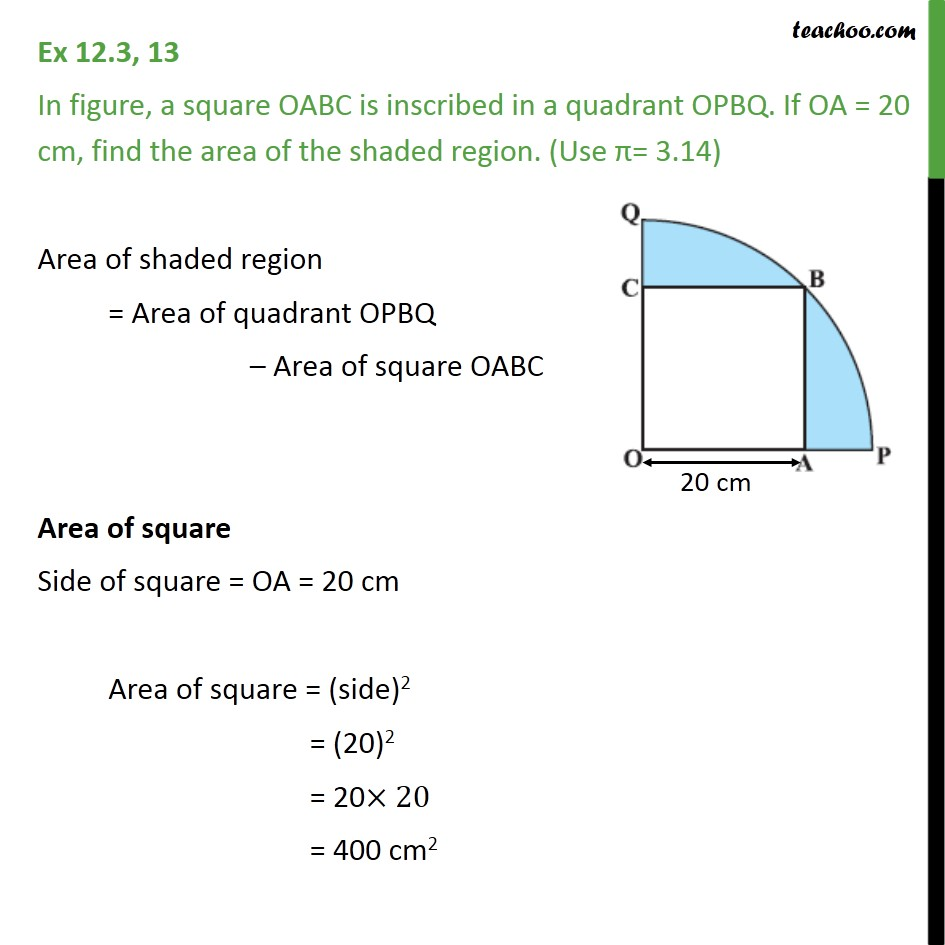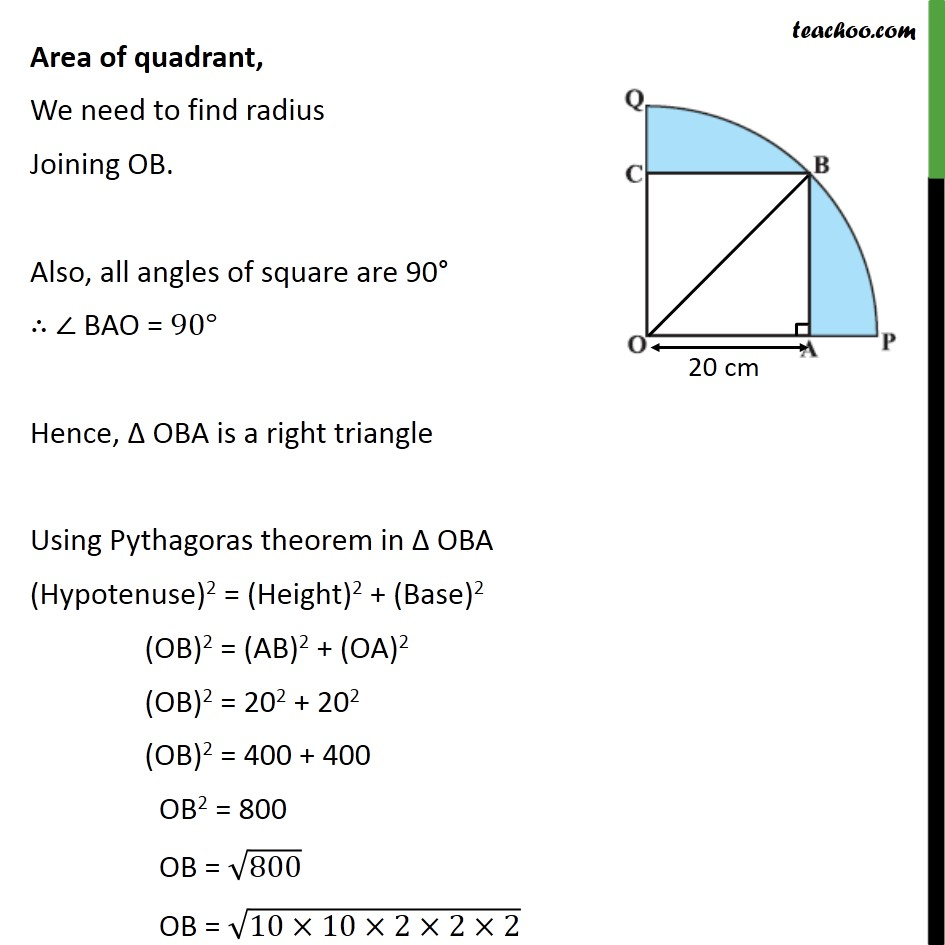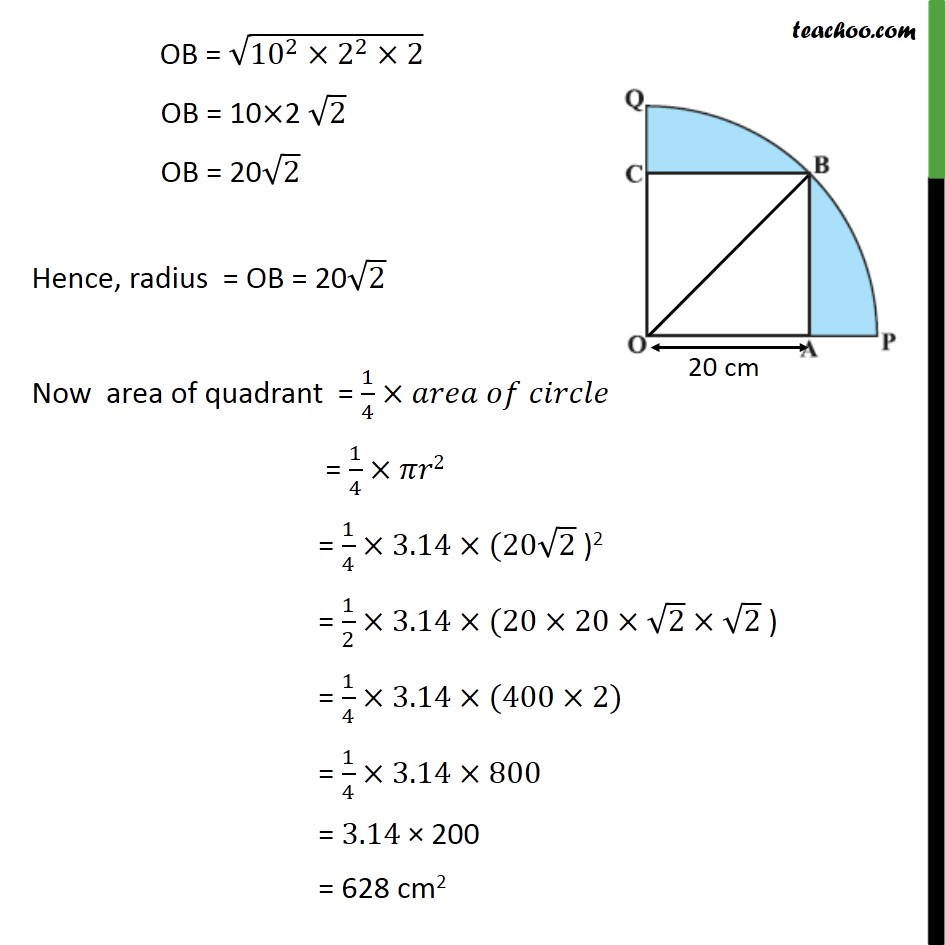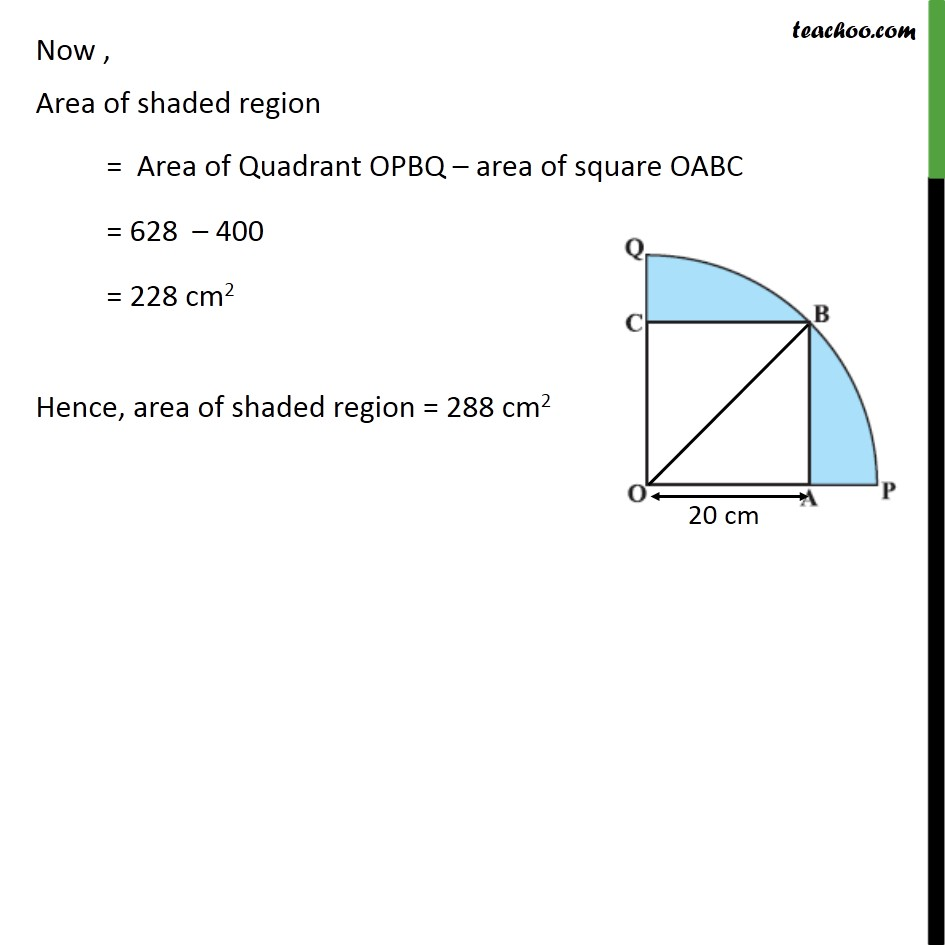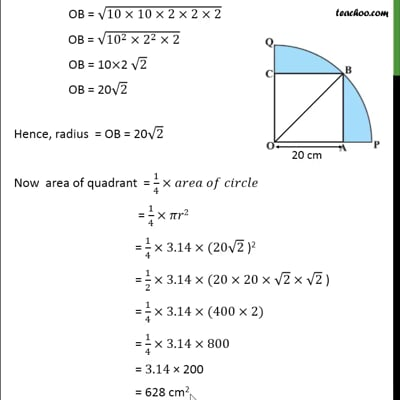This video is only available for Teachoo black users

Solve all your doubts with Teachoo Black (new monthly pack available now!)

### Transcript

Ex 12.3, 13 In figure, a square OABC is inscribed in a quadrant OPBQ. If OA = 20 cm, find the area of the shaded region. (Use π= 3.14) Area of shaded region = Area of quadrant OPBQ – Area of square OABC Area of square Side of square = OA = 20 cm Area of square = (side)2 = (20)2 = 20×20 = 400 cm2 Area of quadrant, We need to find radius Joining OB. Also, all angles of square are 90° ∴ ∠ BAO = 90° Hence, Δ OBA is a right triangle Using Pythagoras theorem in Δ OBA (Hypotenuse)2 = (Height)2 + (Base)2 (OB)2 = (AB)2 + (OA)2 (OB)2 = 202 + 202 (OB)2 = 400 + 400 OB2 = 800 OB = √800 OB = √(10×10×2×2×2) OB = √(〖10〗^2×2^2×2) OB = 10×2 √2 OB = 20√2 Hence, radius = OB = 20√2 Now area of quadrant = 1/4×𝑎𝑟𝑒𝑎 𝑜𝑓 𝑐𝑖𝑟𝑐𝑙𝑒 = 1/4×𝜋𝑟2 = 1/4×3.14×(20√2 )2 = 1/2×3.14×(20×20×√2×√2 ) = 1/4×3.14×(400×2) = 1/4×3.14×800 = 3.14 × 200 = 628 cm2 Now , Area of shaded region = Area of Quadrant OPBQ – area of square OABC = 628 – 400 = 228 cm2 Hence, area of shaded region = 288 cm2